Ex 6.1

Chapter 6 Class 12 Application of Derivatives
Serial order wise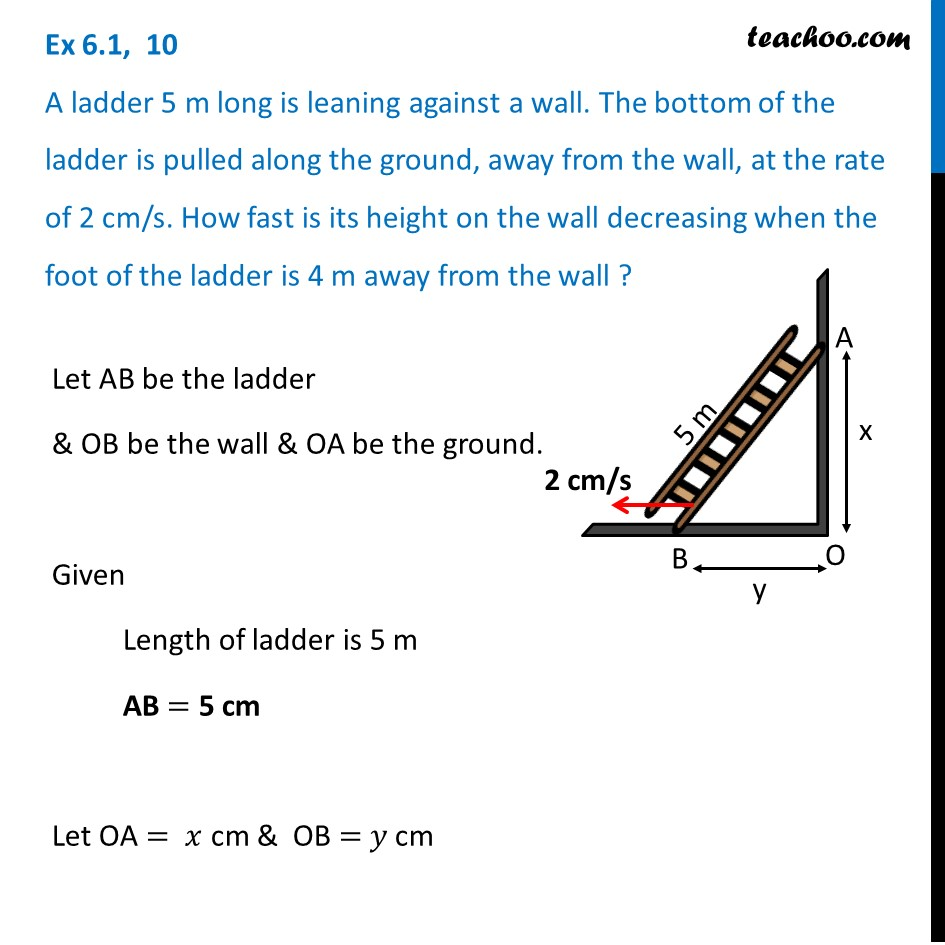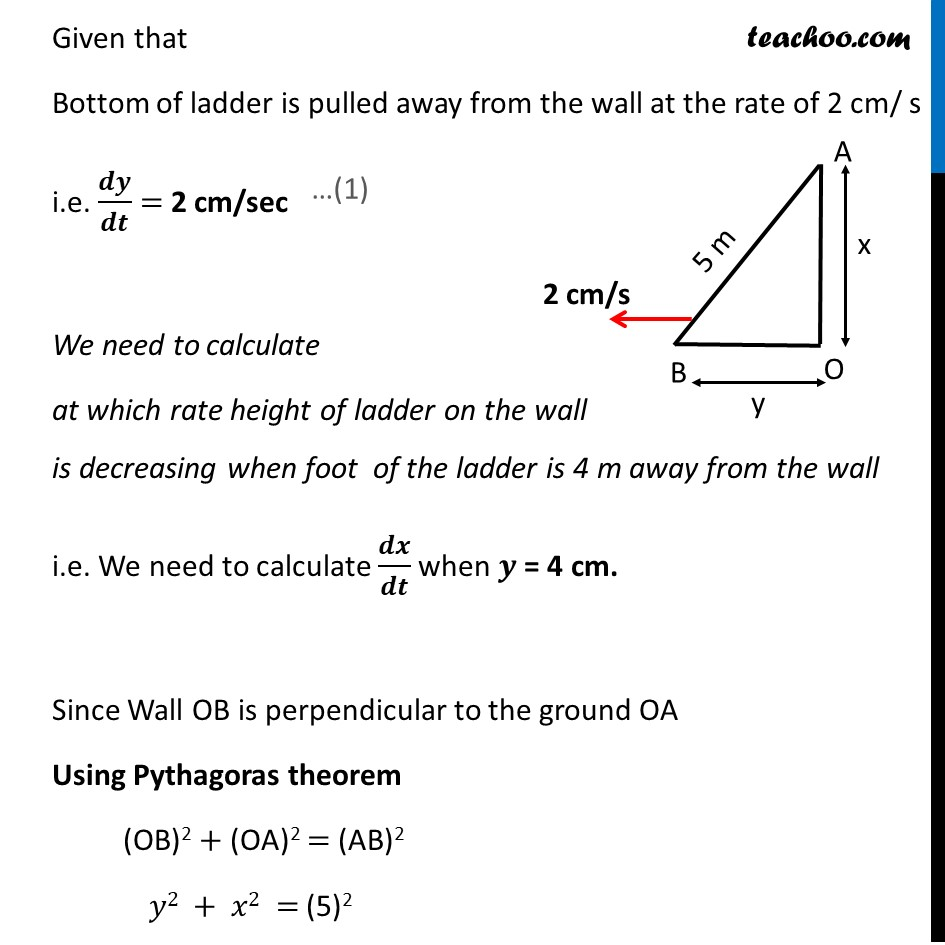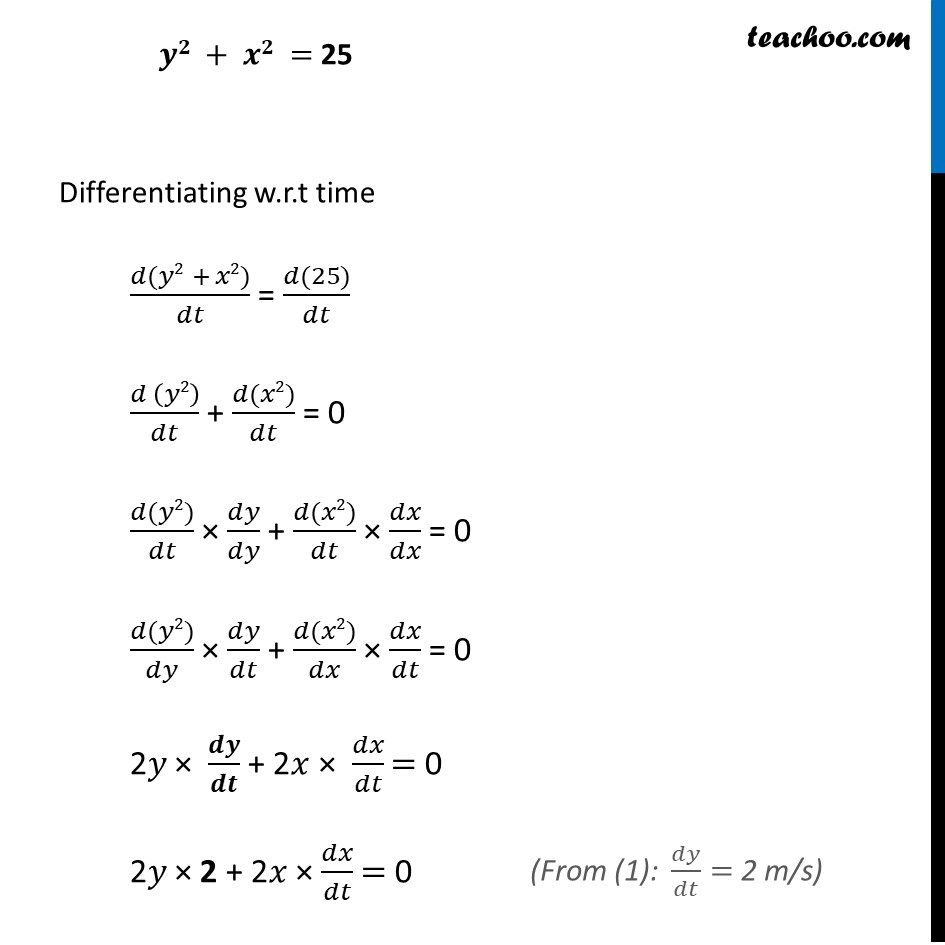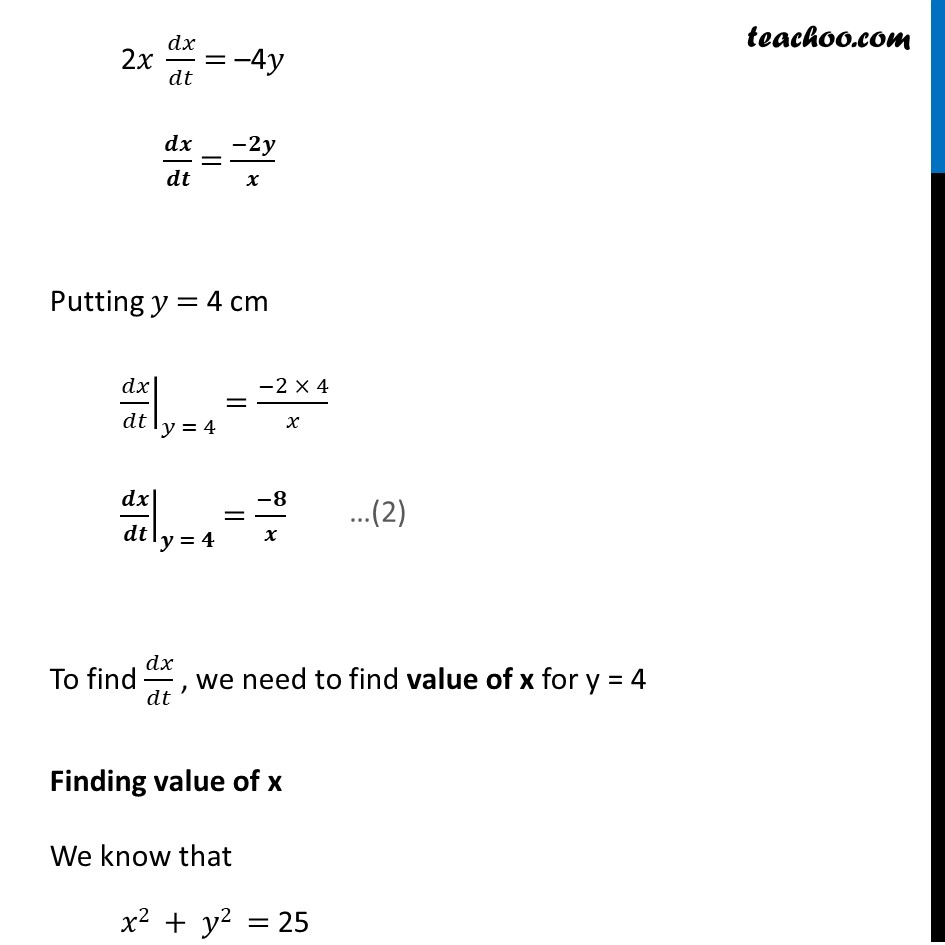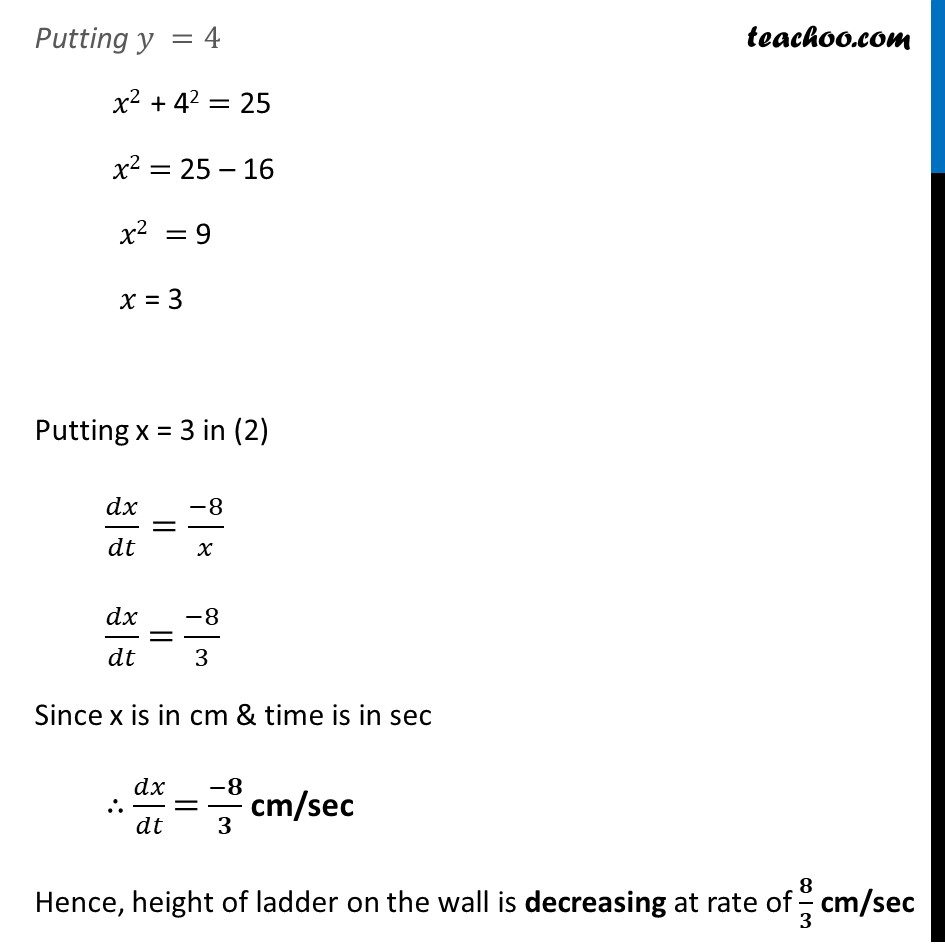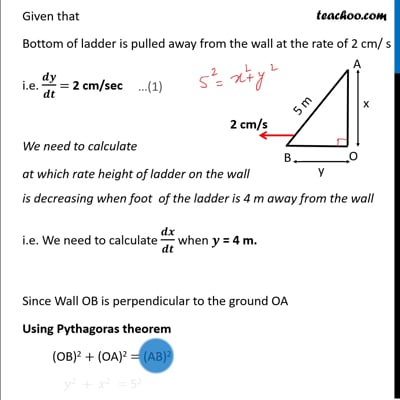This video is only available for Teachoo black users

Maths Crash Course - Live lectures + all videos + Real time Doubt solving!

### Transcript

Ex 6.1, 10 A ladder 5 m long is leaning against a wall. The bottom of the ladder is pulled along the ground, away from the wall, at the rate of 2 cm/s. How fast is its height on the wall decreasing when the foot of the ladder is 4 m away from the wall ?Let AB be the ladder & OB be the wall & OA be the ground. Given Length of ladder is 5 m AB = 5 cm Let OA = 𝑥 cm & OB = 𝑦 cm Given that Bottom of ladder is pulled away from the wall at the rate of 2 cm/ s i.e. 𝒅𝒚/𝒅𝒕 = 2 cm/sec We need to calculate at which rate height of ladder on the wall is decreasing when foot of the ladder is 4 m away from the wall i.e. We need to calculate 𝒅𝒙/𝒅𝒕 when 𝒚 = 4 cm. Since Wall OB is perpendicular to the ground OA Using Pythagoras theorem (OB)2 + (OA)2 = (AB)2 𝑦2 + 𝑥2 = (5)2 𝒚𝟐 + 𝒙𝟐 = 25 Differentiating w.r.t time (𝑑(𝑦2 + 𝑥2))/𝑑𝑡 = (𝑑(25))/𝑑𝑡 (𝑑 (𝑦2))/𝑑𝑡 + (𝑑(𝑥2))/𝑑𝑡 = 0 (𝑑(𝑦2))/𝑑𝑡 × 𝑑𝑦/𝑑𝑦 + (𝑑(𝑥2))/𝑑𝑡 × 𝑑𝑥/𝑑𝑥 = 0 (𝑑(𝑦2))/𝑑𝑦 × 𝑑𝑦/𝑑𝑡 + (𝑑(𝑥2))/𝑑𝑥 × 𝑑𝑥/𝑑𝑡 = 0 2𝑦 × 𝒅𝒚/𝒅𝒕 + 2𝑥 × 𝑑𝑥/𝑑𝑡 = 0 2𝑦 × 2 + 2𝑥 × 𝑑𝑥/𝑑𝑡 = 0 ("From (1): " 𝑑𝑦/𝑑𝑡= 2 m/s) 2𝑥 𝑑𝑥/𝑑𝑡 = –4𝑦 𝒅𝒙/𝒅𝒕 = (−𝟐𝒚)/𝒙 Putting 𝑦 = 4 cm ├ 𝑑𝑥/𝑑𝑡┤|_(𝑦 = 4) =(−2 × 4)/𝑥 ├ 𝒅𝒙/𝒅𝒕┤|_(𝒚 = 𝟒) =(−𝟖)/𝒙 To find 𝑑𝑥/𝑑𝑡 , we need to find value of x for y = 4 Finding value of x We know that 𝑥2 + 𝑦2 = 25 Putting 𝑦 =4 𝑥2 + 42 = 25 𝑥2 = 25 – 16 𝑥2 = 9 𝑥 = 3 Putting x = 3 in (2) 𝑑𝑥/𝑑𝑡=(−8)/𝑥 𝑑𝑥/𝑑𝑡 = (−8)/3 Since x is in cm & time is in sec ∴ 𝑑𝑥/𝑑𝑡 = (−𝟖)/𝟑 cm/sec Hence, height of ladder on the wall is decreasing at rate of 𝟖/𝟑 cm/sec